Data Science and Databases

# Clustering Algorithms: From Start To State Of The Art

Lovro is a Machine Learning Engineer and Data Scientist. He worked at Amazon as well as a researcher at multiple academic institutions.

It’s not a bad time to be a Data Scientist. Serious people may find interest in you if you turn the conversation towards “Big Data”, and the rest of the party crowd will be intrigued when you mention “Artificial Intelligence” and “Machine Learning”. Even Google thinks you’re not bad, and that you’re getting even better. There are a lot of ‘smart’ algorithms that help data scientists do their wizardry. It may all seem complicated, but if we understand and organize algorithms a bit, it’s not even that hard to find and apply the one that we need.

Courses on data mining or machine learning will usually start with clustering, because it is both simple and useful. It is an important part of a somewhat wider area of Unsupervised Learning, where the data we want to describe is not labeled. In most cases this is where the user did not give us much information of what is the expected output. The algorithm only has the data and it should do the best it can. In our case, it should perform clustering – separating data into groups (clusters) that contain similar data points, while the dissimilarity between groups is as high as possible. Data points can represent anything, such as our clients. Clustering can be useful if we, for example, want to group similar users and then run different marketing campaigns on each cluster.

## K-Means Clustering

After the necessary introduction, Data Mining courses always continue with K-Means; an effective, widely used, all-around clustering algorithm. Before actually running it, we have to define a distance function between data points (for example, Euclidean distance if we want to cluster points in space), and we have to set the number of clusters we want (k).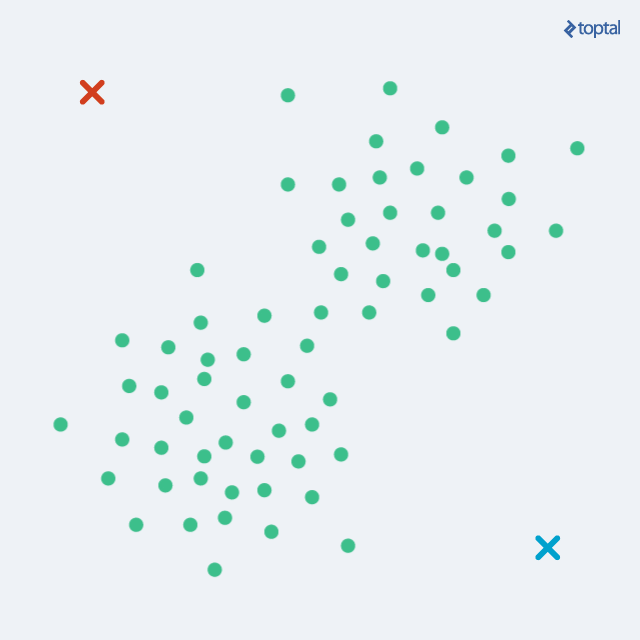The algorithm begins by selecting k points as starting centroids (‘centers’ of clusters). We can just select any k random points, or we can use some other approach, but picking random points is a good start. Then, we iteratively repeat two steps:

1. Assignment step: each of m points from our dataset is assigned to a cluster that is represented by the closest of the k centroids. For each point, we calculate distances to each centroid, and simply pick the least distant one.

2. Update step: for each cluster, a new centroid is calculated as the mean of all points in the cluster. From the previous step, we have a set of points which are assigned to a cluster. Now, for each such set, we calculate a mean that we declare a new centroid of the cluster.

After each iteration, the centroids are slowly moving, and the total distance from each point to its assigned centroid gets lower and lower. The two steps are alternated until convergence, meaning until there are no more changes in cluster assignment. After a number of iterations, the same set of points will be assigned to each centroid, therefore leading to the same centroids again. K-Means is guaranteed to converge to a local optimum. However, that does not necessarily have to be the best overall solution (global optimum).

The final clustering result can depend on the selection of initial centroids, so a lot of thought has been given to this problem. One simple solution is just to run K-Means a couple of times with random initial assignments. We can then select the best result by taking the one with the minimal sum of distances from each point to its cluster – the error value that we are trying to minimize in the first place.

Other approaches to selecting initial points can rely on selecting distant points. This can lead to better results, but we may have a problem with outliers, those rare alone points that are just “off” that may just be some errors. Since they are far from any meaningful cluster, each such point may end up being its own ‘cluster’. A good balance is K-Means++ variant [Arthur and Vassilvitskii, 2007], whose initialization will still pick random points, but with probability proportional to square distance from the previously assigned centroids. Points that are further away will have higher probability to be selected as starting centroids. Consequently, if there’s a group of points, the probability that a point from the group will be selected also gets higher as their probabilities add up, resolving the outlier problem we mentioned.

K-Means++ is also the default initialization for Python’s Scikit-learn K-Means implementation. If you’re using Python, this may be your library of choice. For Java, Weka library may be a good start:

#### Java (Weka)

``````// Load some data

// Create the model
SimpleKMeans kMeans = new SimpleKMeans();

// We want three clusters
kMeans.setNumClusters(3);

// Run K-Means
kMeans.buildClusterer(data);

// Print the centroids
Instances centroids = kMeans.getClusterCentroids();
for (Instance centroid: centroids) {
System.out.println(centroid);
}

// Print cluster membership for each instance
for (Instance point: data) {
System.out.println(point + " is in cluster " + kMeans.clusterInstance(point));
}
``````

#### Python (Scikit-learn)

``````> >> from sklearn import cluster, datasets
> >> Xiris = iris.data
> >> yiris = iris.target

> >> kmeans = cluster.KMeans(nclusters=3)
> >> kmeans.fit(Xiris)
KMeans(copy_x=True, init='k-means++', ...
> >> print(kmeans.labels[::10])
[1 1 1 1 1 0 0 0 0 0 2 2 2 2 2]
> >> print(yiris[::10])
[0 0 0 0 0 1 1 1 1 1 2 2 2 2 2]
``````

In the Python example above, we used a standard example dataset ‘Iris’, which contains flower petal and sepal dimensions for three different species of iris. We clustered these into three clusters, and compared the obtained clusters to the actual species (target), to see that they match perfectly.

In this case, we knew that there were three different clusters (species), and K-Means recognized correctly which ones go together. But, how do we choose a good number of clusters k in general? These kind of questions are quite common in Machine Learning. If we request more clusters, they will be smaller, and therefore the total error (total of distances from points to their assigned clusters) will be smaller. So, is it a good idea just to set a bigger k? We may end with having k = m, that is, each point being its own centroid, with each cluster having only one point. Yes, the total error is 0, but we didn’t get a simpler description of our data, nor is it general enough to cover some new points that may appear. This is called overfitting, and we don’t want that.

A way to deal with this problem is to include some penalty for a larger number of clusters. So, we are now trying to minimize not only the error, but error + penalty. The error will just converge towards zero as we increase the number of clusters, but the penalty will grow. At some points, the gain from adding another cluster will be less than the introduced penalty, and we’ll have the optimal result. A solution that uses Bayesian Information Criterion (BIC) for this purpose is called X-Means [Pelleg and Moore, 2000].

Another thing we have to define properly is the distance function. Sometimes that’s a straightforward task, a logical one given the nature of data. For points in space, Euclidean distance is an obvious solution, but it may be tricky for features of different ‘units’, for discrete variables, etc. This may require a lot of domain knowledge. Or, we can call Machine Learning for help. We can actually try to learn the distance function. If we have a training set of points that we know how they should be grouped (i.e. points labeled with their clusters), we can use supervised learning techniques to find a good function, and then apply it to our target set that is not yet clustered.

The method used in K-Means, with its two alternating steps resembles an Expectation–Maximization (EM) method. Actually, it can be considered a very simple version of EM. However, it should not be confused with the more elaborate EM clustering algorithm even though it shares some of the same principles.

## EM Clustering

So, with K-Means clustering each point is assigned to just a single cluster, and a cluster is described only by its centroid. This is not too flexible, as we may have problems with clusters that are overlapping, or ones that are not of circular shape. With EM Clustering, we can now go a step further and describe each cluster by its centroid (mean), covariance (so that we can have elliptical clusters), and weight (the size of the cluster). The probability that a point belongs to a cluster is now given by a multivariate Gaussian probability distribution (multivariate - depending on multiple variables). That also means that we can calculate the probability of a point being under a Gaussian ‘bell’, i.e. the probability of a point belonging to a cluster.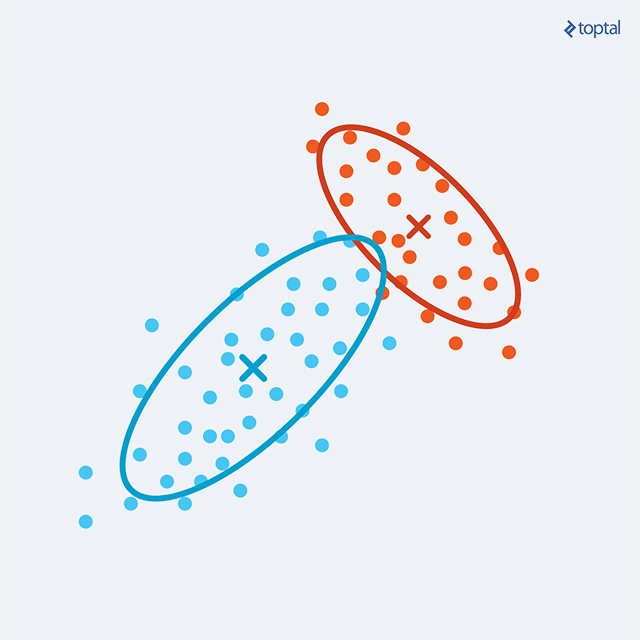We now start the EM procedure by calculating, for each point, the probabilities of it belonging to each of the current clusters (which, again, may be randomly created at the beginning). This is the E-step. If one cluster is a really good candidate for a point, it will have a probability close to one. However, two or more clusters can be acceptable candidates, so the point has a distribution of probabilities over clusters. This property of the algorithm, of points not belonging restricted to one cluster is called “soft clustering”.

The M-step now recalculates the parameters of each cluster, using the assignments of points to the previous set of clusters. To calculate the new mean, covariance and weight of a cluster, each point data is weighted by its probability of belonging to the cluster, as calculated in the previous step.

Alternating these two steps will increase the total log-likelihood until it converges. Again, the maximum may be local, so we can run the algorithm several times to get better clusters.

If we now want to determine a single cluster for each point, we may simply choose the most probable one. Having a probability model, we can also use it to generate similar data, that is to sample more points that are similar to the data that we observed.

## Affinity Propagation

Affinity Propagation (AP) was published by Frey and Dueck in 2007, and is only getting more and more popular due to its simplicity, general applicability, and performance. It is changing its status from state of the art to de facto standard.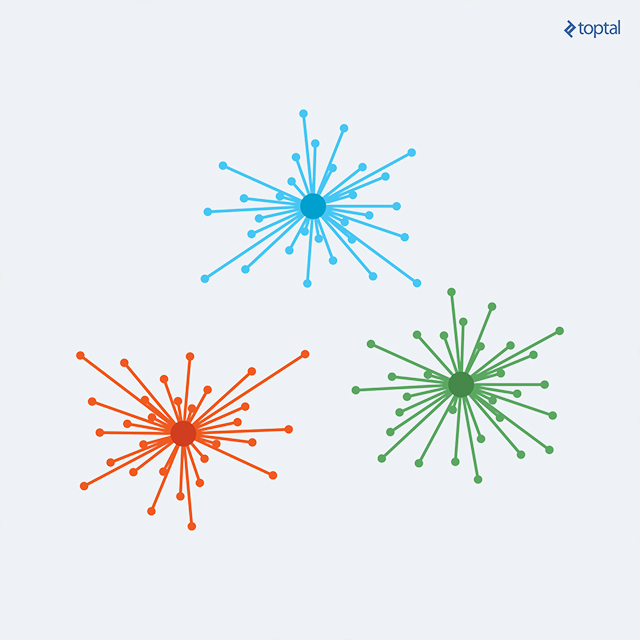The main drawbacks of K-Means and similar algorithms are having to select the number of clusters, and choosing the initial set of points. Affinity Propagation, instead, takes as input measures of similarity between pairs of data points, and simultaneously considers all data points as potential exemplars. Real-valued messages are exchanged between data points until a high-quality set of exemplars and corresponding clusters gradually emerges.

As an input, the algorithm requires us to provide two sets of data:

1. Similarities between data points, representing how well-suited a point is to be another one’s exemplar. If there’s no similarity between two points, as in they cannot belong to the same cluster, this similarity can be omitted or set to -Infinity depending on implementation.

2. Preferences, representing each data point’s suitability to be an exemplar. We may have some a priori information which points could be favored for this role, and so we can represent it through preferences.

Both similarities and preferences are often represented through a single matrix, where the values on the main diagonal represent preferences. Matrix representation is good for dense datasets. Where connections between points are sparse, it is more practical not to store the whole n x n matrix in memory, but instead keep a list of similarities to connected points. Behind the scene, ‘exchanging messages between points’ is the same thing as manipulating matrices, and it’s only a matter of perspective and implementation.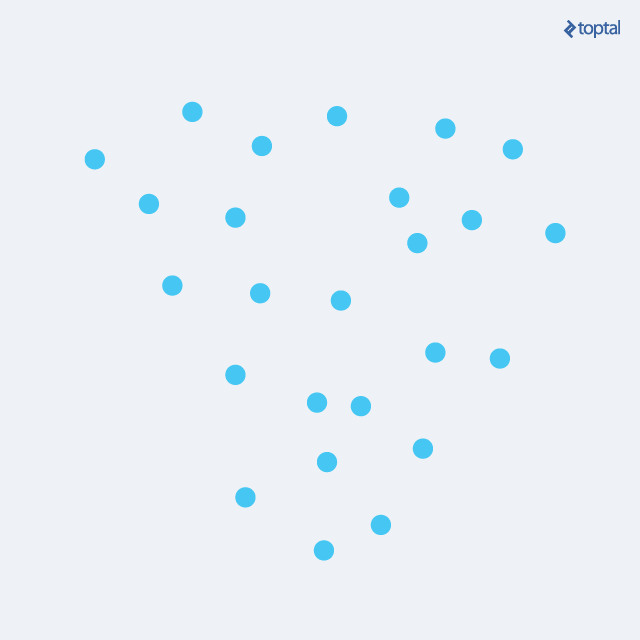The algorithm then runs through a number of iterations, until it converges. Each iteration has two message-passing steps:

1. Calculating responsibilities: Responsibility r(i, k) reflects the accumulated evidence for how well-suited point k is to serve as the exemplar for point i, taking into account other potential exemplars for point i. Responsibility is sent from data point i to candidate exemplar point k.

2. Calculating availabilities: Availability a(i, k) reflects the accumulated evidence for how appropriate it would be for point i to choose point k as its exemplar, taking into account the support from other points that point k should be an exemplar. Availability is sent from candidate exemplar point k to point i.

In order to calculate responsibilities, the algorithm uses original similarities and availabilities calculated in the previous iteration (initially, all availabilities are set to zero). Responsibilities are set to the input similarity between point i and point k as its exemplar, minus the largest of the similarity and availability sum between point i and other candidate exemplars. The logic behind calculating how suitable a point is for an exemplar is that it is favored more if the initial a priori preference was higher, but the responsibility gets lower when there is a similar point that considers itself a good candidate, so there is a ‘competition’ between the two until one is decided in some iteration.

Calculating availabilities, then, uses calculated responsibilities as evidence whether each candidate would make a good exemplar. Availability a(i, k) is set to the self-responsibility r(k, k) plus the sum of the positive responsibilities that candidate exemplar k receives from other points.

Finally, we can have different stopping criteria to terminate the procedure, such as when changes in values fall below some threshold, or the maximum number of iterations is reached. At any point through Affinity Propagation procedure, summing Responsibility (r) and Availability (a) matrices gives us the clustering information we need: for point i, the k with maximum r(i, k) + a(i, k) represents point i’s exemplar. Or, if we just need the set of exemplars, we can scan the main diagonal. If r(i, i) + a(i, i) > 0, point i is an exemplar.

We’ve seen that with K-Means and similar algorithms, deciding the number of clusters can be tricky. With AP, we don’t have to explicitly specify it, but it may still need some tuning if we obtain either more or less clusters than we may find optimal. Luckily, just by adjusting the preferences we can lower or raise the number of clusters. Setting preferences to a higher value will lead to more clusters, as each point is ‘more certain’ of its suitability to be an exemplar and is therefore harder to ‘beat’ and include it under some other point’s ‘domination’. Conversely, setting lower preferences will result in having less clusters; as if they’re saying “no, no, please, you’re a better exemplar, I’ll join your cluster”. As a general rule, we may set all preferences to the median similarity for a medium to large number of clusters, or to the lowest similarity for a moderate number of clusters. However, a couple of runs with adjusting preferences may be needed to get the result that exactly suits our needs.

Hierarchical Affinity Propagation is also worth mentioning, as a variant of the algorithm that deals with quadratic complexity by splitting the dataset into a couple of subsets, clustering them separately, and then performing the second level of clustering.

## In The End…

There’s a whole range of clustering algorithms, each one with its pros and cons regarding what type of data they with, time complexity, weaknesses, and so on. To mention more algorithms, for example there’s Hierarchical Agglomerative Clustering (or Linkage Clustering), good for when we don’t necessarily have circular (or hyper-spherical) clusters, and don’t know the number of clusters in advance. It starts with each point being a separate cluster, and works by joining two closest clusters in each step until everything is in one big cluster.

With Hierarchical Agglomerative Clustering, we can easily decide the number of clusters afterwards by cutting the dendrogram (tree diagram) horizontally where we find suitable. It is also repeatable (always gives the same answer for the same dataset), but is also of a higher complexity (quadratic).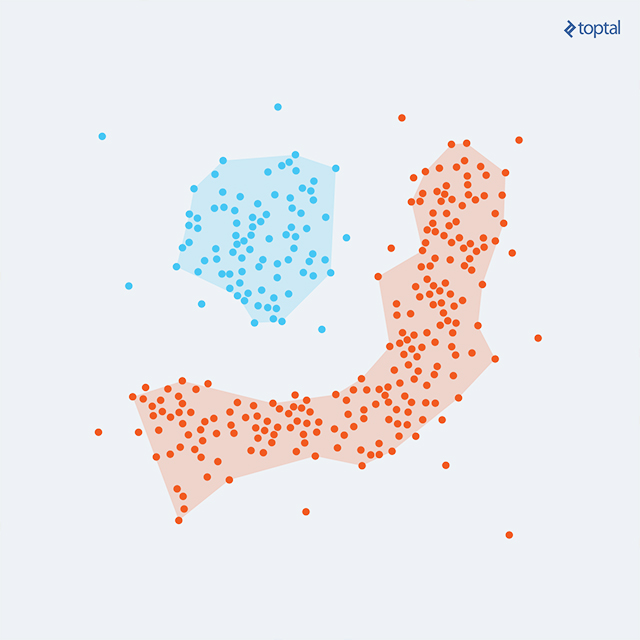Then, DBSCAN (Density-Based Spatial Clustering of Applications with Noise) is also an algorithm worth mentioning. It groups points that are closely packed together, expanding clusters in any direction where there are nearby points, thus dealing with different shapes of clusters.

These algorithms deserve an article of their own, and we can explore them in a separate post later on.

It takes experience with some trial and error to know when to use one algorithm or the other. Luckily, we have a range of implementations in different programming languages, so trying them out only requires a little willingness to play.